27.2 Huygens's principle: diffraction

 Page 1 / 3
• Discuss the propagation of transverse waves.
• Discuss Huygens’s principle.
• Explain the bending of light.

[link] shows how a transverse wave looks as viewed from above and from the side. A light wave can be imagined to propagate like this, although we do not actually see it wiggling through space. From above, we view the wavefronts (or wave crests) as we would by looking down on the ocean waves. The side view would be a graph of the electric or magnetic field. The view from above is perhaps the most useful in developing concepts about wave optics.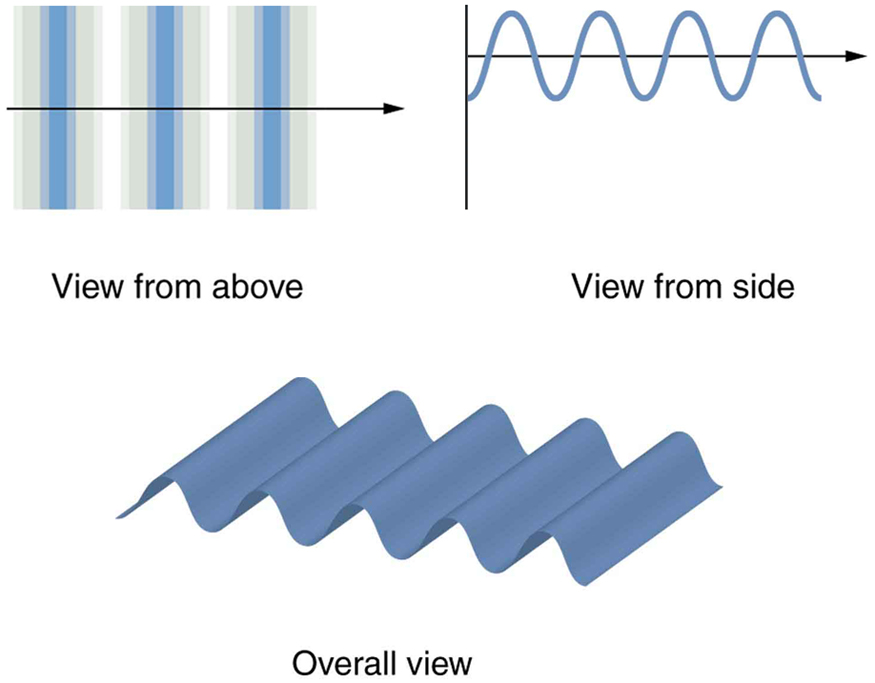A transverse wave, such as an electromagnetic wave like light, as viewed from above and from the side. The direction of propagation is perpendicular to the wavefronts (or wave crests) and is represented by an arrow like a ray.

The Dutch scientist Christiaan Huygens (1629–1695) developed a useful technique for determining in detail how and where waves propagate. Starting from some known position, Huygens’s principle    states that:

Every point on a wavefront is a source of wavelets that spread out in the forward direction at the same speed as the wave itself. The new wavefront is a line tangent to all of the wavelets.

[link] shows how Huygens’s principle is applied. A wavefront is the long edge that moves, for example, the crest or the trough. Each point on the wavefront emits a semicircular wave that moves at the propagation speed $v$ . These are drawn at a time $t$ later, so that they have moved a distance $s=\text{vt}$ . The new wavefront is a line tangent to the wavelets and is where we would expect the wave to be a time $t$ later. Huygens’s principle works for all types of waves, including water waves, sound waves, and light waves. We will find it useful not only in describing how light waves propagate, but also in explaining the laws of reflection and refraction. In addition, we will see that Huygens’s principle tells us how and where light rays interfere.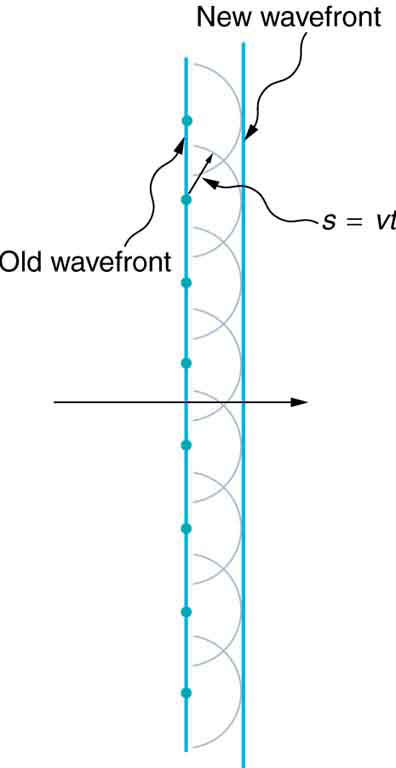Huygens’s principle applied to a straight wavefront. Each point on the wavefront emits a semicircular wavelet that moves a distance s = v t . The new wavefront is a line tangent to the wavelets.

[link] shows how a mirror reflects an incoming wave at an angle equal to the incident angle, verifying the law of reflection. As the wavefront strikes the mirror, wavelets are first emitted from the left part of the mirror and then the right. The wavelets closer to the left have had time to travel farther, producing a wavefront traveling in the direction shown.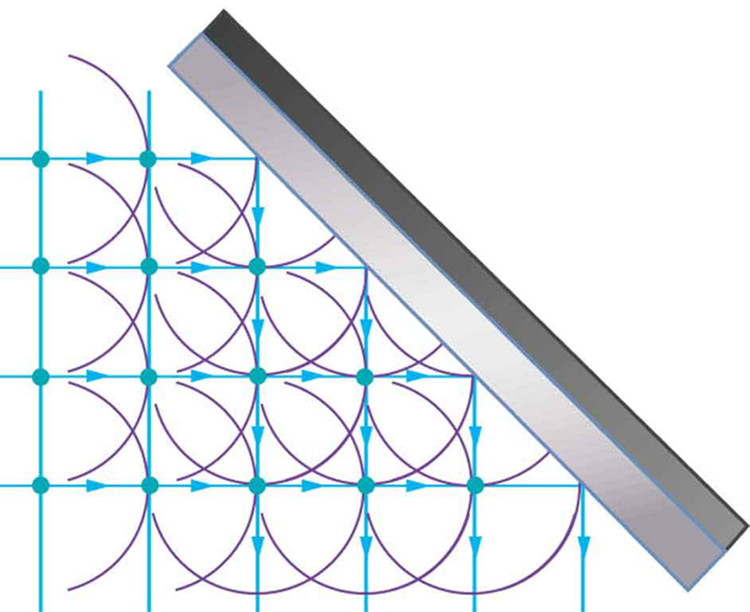Huygens’s principle applied to a straight wavefront striking a mirror. The wavelets shown were emitted as each point on the wavefront struck the mirror. The tangent to these wavelets shows that the new wavefront has been reflected at an angle equal to the incident angle. The direction of propagation is perpendicular to the wavefront, as shown by the downward-pointing arrows.

The law of refraction can be explained by applying Huygens’s principle to a wavefront passing from one medium to another (see [link] ). Each wavelet in the figure was emitted when the wavefront crossed the interface between the media. Since the speed of light is smaller in the second medium, the waves do not travel as far in a given time, and the new wavefront changes direction as shown. This explains why a ray changes direction to become closer to the perpendicular when light slows down. Snell’s law can be derived from the geometry in [link] , but this is left as an exercise for ambitious readers.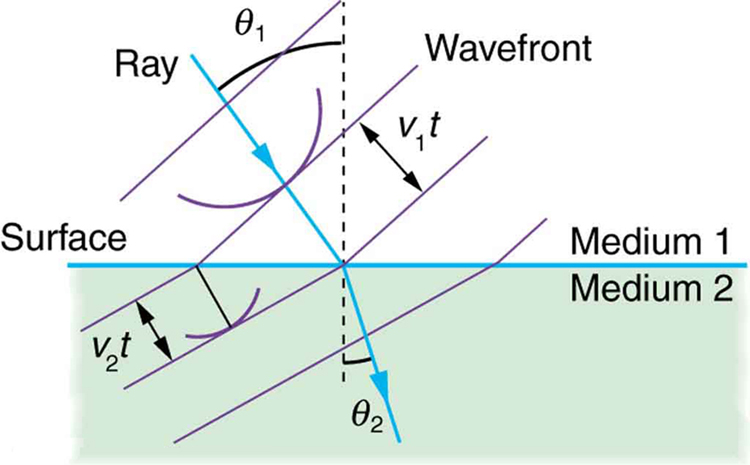Huygens’s principle applied to a straight wavefront traveling from one medium to another where its speed is less. The ray bends toward the perpendicular, since the wavelets have a lower speed in the second medium.

What happens when a wave passes through an opening, such as light shining through an open door into a dark room? For light, we expect to see a sharp shadow of the doorway on the floor of the room, and we expect no light to bend around corners into other parts of the room. When sound passes through a door, we expect to hear it everywhere in the room and, thus, expect that sound spreads out when passing through such an opening (see [link] ). What is the difference between the behavior of sound waves and light waves in this case? The answer is that light has very short wavelengths and acts like a ray. Sound has wavelengths on the order of the size of the door and bends around corners (for frequency of 1000 Hz, $\lambda =c/f=\left(\text{330}\phantom{\rule{0.25em}{0ex}}\text{m}/\text{s}\right)/\left(\text{1000}\phantom{\rule{0.25em}{0ex}}{\text{s}}^{-1}\right)=0\text{.}\text{33 m}$ , about three times smaller than the width of the doorway).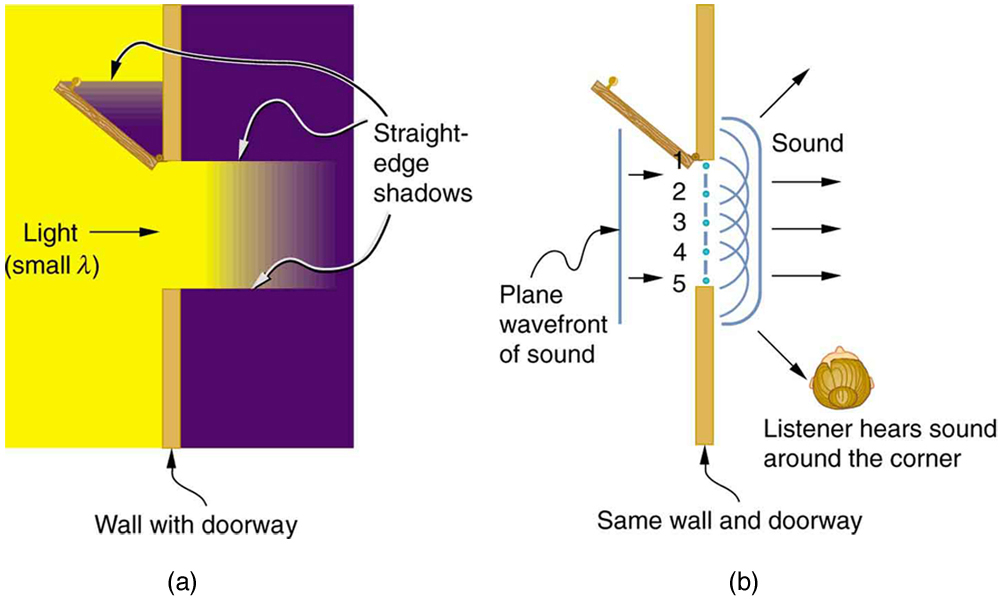(a) Light passing through a doorway makes a sharp outline on the floor. Since light’s wavelength is very small compared with the size of the door, it acts like a ray. (b) Sound waves bend into all parts of the room, a wave effect, because their wavelength is similar to the size of the door.

If we pass light through smaller openings, often called slits, we can use Huygens’s principle to see that light bends as sound does (see [link] ). The bending of a wave around the edges of an opening or an obstacle is called diffraction    . Diffraction is a wave characteristic and occurs for all types of waves. If diffraction is observed for some phenomenon, it is evidence that the phenomenon is a wave. Thus the horizontal diffraction of the laser beam after it passes through slits in [link] is evidence that light is a wave.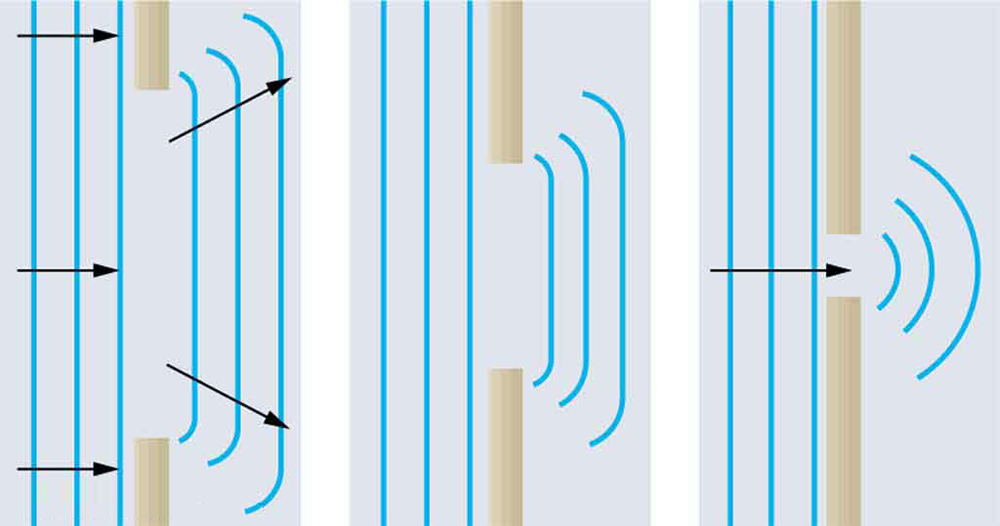Huygens’s principle applied to a straight wavefront striking an opening. The edges of the wavefront bend after passing through the opening, a process called diffraction. The amount of bending is more extreme for a small opening, consistent with the fact that wave characteristics are most noticeable for interactions with objects about the same size as the wavelength.

Section summary

• An accurate technique for determining how and where waves propagate is given by Huygens’s principle: Every point on a wavefront is a source of wavelets that spread out in the forward direction at the same speed as the wave itself. The new wavefront is a line tangent to all of the wavelets.
• Diffraction is the bending of a wave around the edges of an opening or other obstacle.

Conceptual questions

How do wave effects depend on the size of the object with which the wave interacts? For example, why does sound bend around the corner of a building while light does not?

Under what conditions can light be modeled like a ray? Like a wave?

Go outside in the sunlight and observe your shadow. It has fuzzy edges even if you do not. Is this a diffraction effect? Explain.

Why does the wavelength of light decrease when it passes from vacuum into a medium? State which attributes change and which stay the same and, thus, require the wavelength to decrease.

Does Huygens’s principle apply to all types of waves?

Calculate the work done by an 85.0-kg man who pushes a crate 4.00 m up along a ramp that makes an angle of 20.0º20.0º with the horizontal. (See [link] .) He exerts a force of 500 N on the crate parallel to the ramp and moves at a constant speed. Be certain to include the work he does on the crate an
What is thermal heat all about
why uniform circular motion is called a periodic motion?.
when a train start from A & it returns at same station A . what is its acceleration?
what is distance of A to B of the stations and what is the time taken to reach B from A
BELLO
the information provided is not enough
aliyu
Hmmmm maybe the question is logical
yusuf
where are the parameters for calculation
HENRY
there is enough information to calculate an AVERAGE acceleration
Kwok
mistake, there is enough information to calculate an average velocity
Kwok
~\
Abel
what is the unit of momentum
Abel
wha are the types of radioactivity ?
what are the types of radioactivity
Worku
what is static friction
It is the opposite of kinetic friction
Mark
static fiction is friction between two surfaces in contact an none of sliding over on another, while Kinetic friction is friction between sliding surfaces in contact.
MINDERIUM
I don't get it,if it's static then there will be no friction.
author
It means that static friction is that friction that most be overcome before a body can move
kingsley
static friction is a force that keeps an object from moving, and it's the opposite of kinetic friction.
author
It is a force a body must overcome in order for the body to move.
Eboh
If a particle accelerator explodes what happens
Eboh
why we see the edge effect in case of the field lines of capacitor?
Arnab
what is wave
what is force
Muhammed
force is something which is responsible for the object to change its position
MINDERIUM
more technically it is the product of mass of an object and Acceleration produced in it
MINDERIUM
wave is disturbance in any medium
iqra
energy is distributed in any medium through particles of medium.
iqra
If a particle accelerator explodes what happens
we have to first figure out .... wats a particle accelerator first
Teh
What is surface tension
The resistive force of surface.
iqra
Who can tutor me on simple harmonic motion
on both a string and peldulum?
Anya
spring*
Anya
Yea
yusuf
Do you have a chit-chat contact
yusuf
I dont have social media but i do have an email?
Anya
Which is
yusuf
Where are you chatting from
yusuf
I don't understand the basics of this group
Jimmy
teach him SHM init
Anya
Simple harmonic motion
yusuf
how.an.equipotential.line is two dimension and equipotential surface is three dimension ?
definition of mass of conversion
Force equals mass time acceleration. Weight is a force and it can replace force in the equation. The acceleration would be gravity, which is an acceleration. To change from weight to mass divide by gravity (9.8 m/s^2).
Marisa
how many subject is in physics
the write question should be " How many Topics are in O- Level Physics, or other branches of physics.
effiom
how many topic are in physics
Praise
Praise what level are you
yusuf
If u are doing a levels in your first year you do AS topics therefore you do 5 big topic i.e particles radiation, waves and optics, mechanics,materials, electricity. After that you do A level topics like Specific Harmonic motion circular motion astrophysics depends really
Anya
Yeah basics of physics prin8
yusuf
Heat nd Co for a level
yusuf
yh I need someone to explain something im tryna solve . I'll send the question if u down for it
a ripple tank experiment a vibrating plane is used to generate wrinkles in the water .if the distance between two successive point is 3.5cm and the wave travel a distance of 31.5cm find the frequency of the vibration
Tamdy
hallow
Boniface
Boniface
the range of objects and phenomena studied in physics is
BonifaceByBy Lakeima RobertsBy Jordon Humphreys